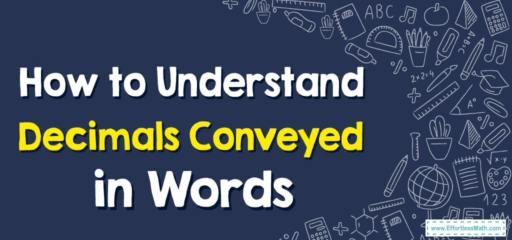# How to Understand Decimals Conveyed in Words

Understanding decimals conveyed in words is an essential skill for numerical literacy. This comprehensive guide takes you through a step-by-step process, from identifying decimal places to using place value words. Enhance your ability to interpret and comprehend decimals expressed in word form with this invaluable resource.## A Step-by-step guide to How to Understand Decimals Conveyed in Words

### Step 1: Familiarize yourself with the decimal places

Decimals are based on a place value system, just like whole numbers. The value of each digit in a decimal decreases from left to right. The first digit to the right of the decimal point represents tenths, the second represents hundredths, the third represents thousandths, and so on.

### Step 2: Read the whole number part

Before you get to the decimal portion, there might be a whole number part that appears before the decimal point. Read it as you would any other whole number.

### Step 3: Identify the decimal point

Locate the decimal point in the given number. This point separates the whole number part from the decimal part.

### Step 4: Begin reading the decimal part

Start reading the decimal portion digit by digit, from left to right. For each digit, state its value based on its position.

### Step 5: Use place value words

As you read each digit in the decimal part, use the appropriate place value word to convey its value. Here are the place value words commonly used:

• Tenths: This represents the first digit to the right of the decimal point. For example, the digit 3 in the decimal 0.3 is read as “three tenths.”
• Hundredths: This represents the second digit to the right of the decimal point. For example, the digit 7 in the decimal 0.37 is read as “seven hundredths.”
• Thousandths: This represents the third digit to the right of the decimal point. For example, the digit 9 in the decimal 0.379 is read as “nine thousandths.”

Continue this pattern for each subsequent digit in the decimal part, assigning the appropriate place value word.

### Step 6: Consider zero placeholders

If there are zero digits in the decimal part, you can still indicate their presence by using the word “zero.” For example, the decimal 0.05 is read as “zero point zero five.”

### Step 7: Wrap up the reading

After you have read all the digits in the decimal part, you can end by indicating the end of the decimal portion. For example, you can say “and” or “point” to signal the end of the decimal.

### Step 8: Combine the whole number and decimal readings

To express the complete number, combine the whole number part (if it exists) with the decimal part. Use the words “and” or “point” to connect the two parts. For example:

• The decimal 3.75 can be read as “three and seventy-five hundredths.”
• The decimal 14.02 can be read as “fourteen and two hundredths.”

By following these steps, you should be able to understand decimals conveyed in words more effectively. Practice reading different decimals aloud to enhance your understanding and fluency.

### What people say about "How to Understand Decimals Conveyed in Words - Effortless Math: We Help Students Learn to LOVE Mathematics"?

No one replied yet.

X
30% OFF

Limited time only!

Save Over 30%

SAVE $5 It was$16.99 now it is \$11.99